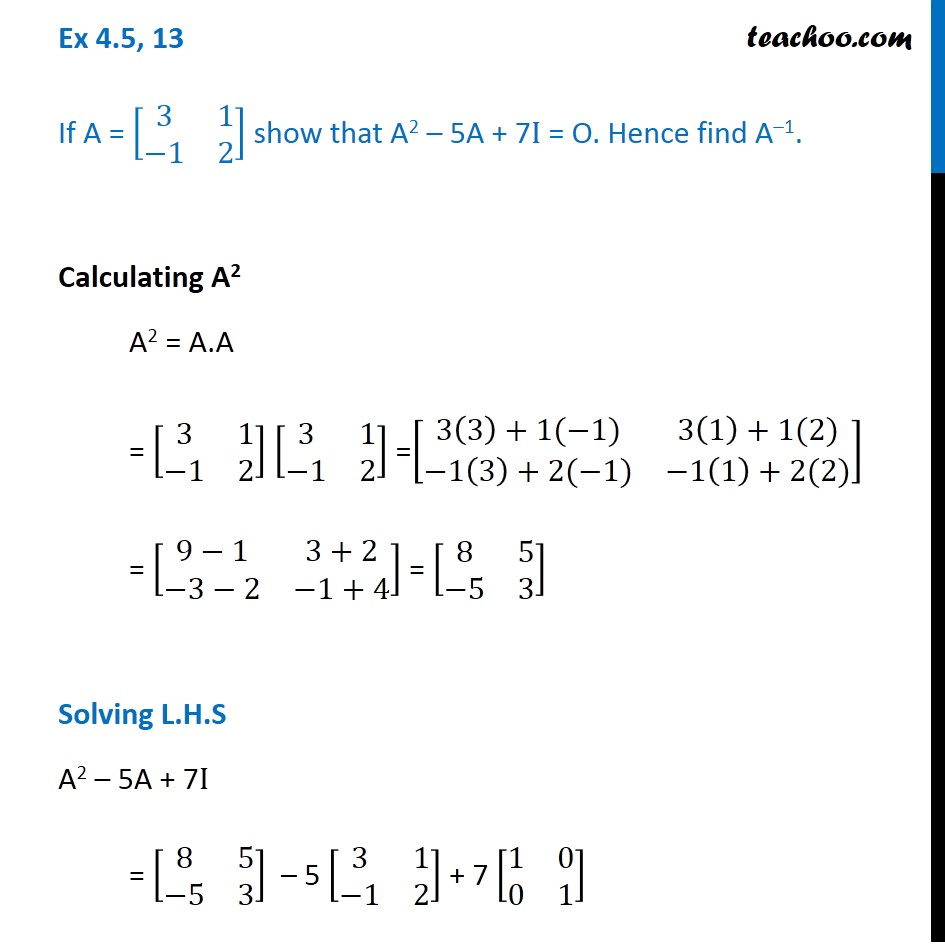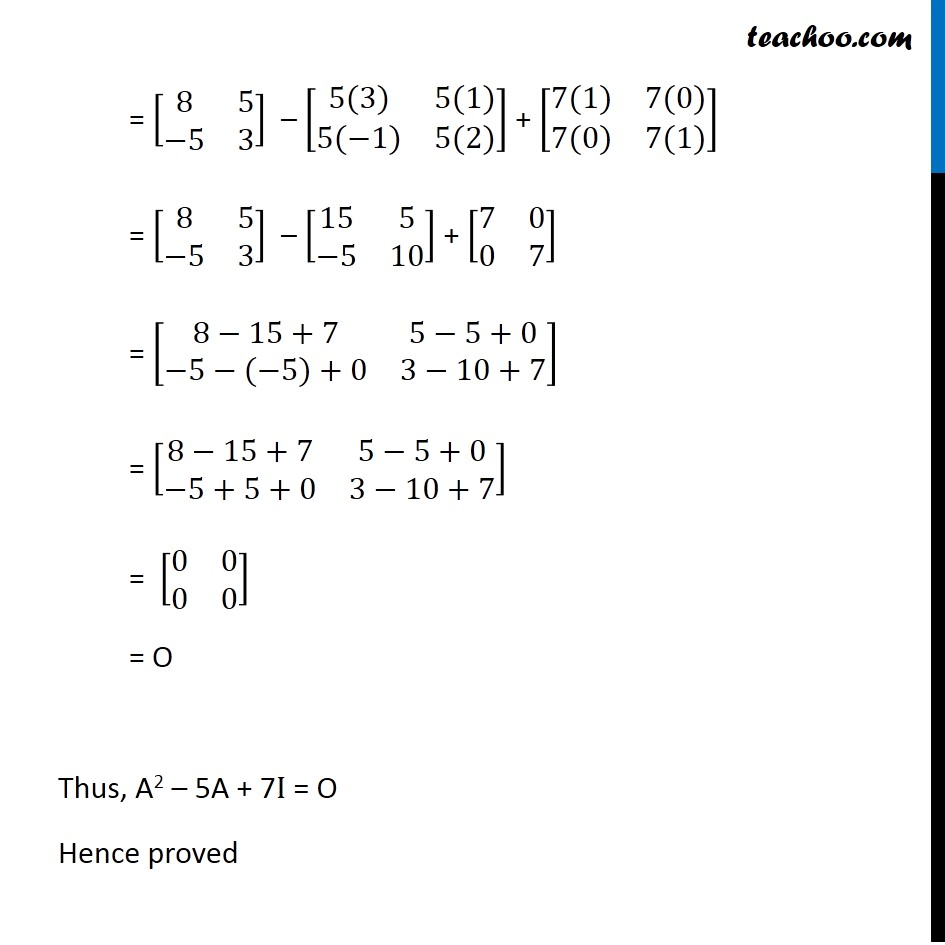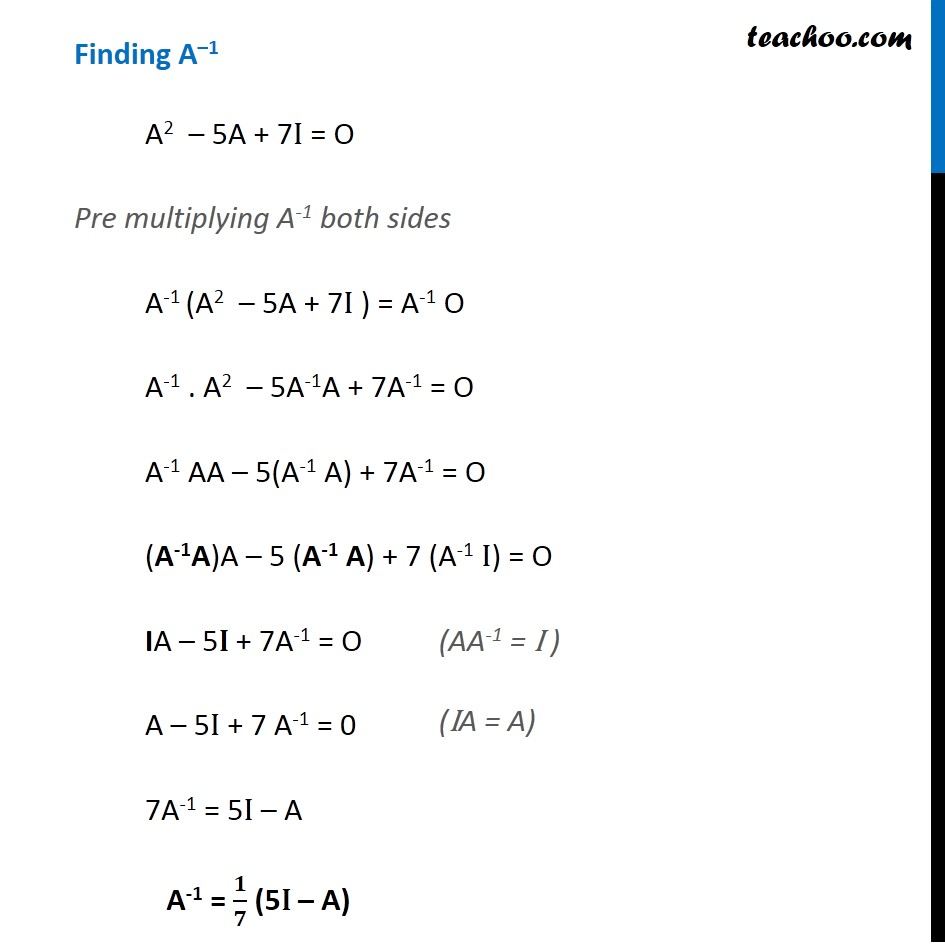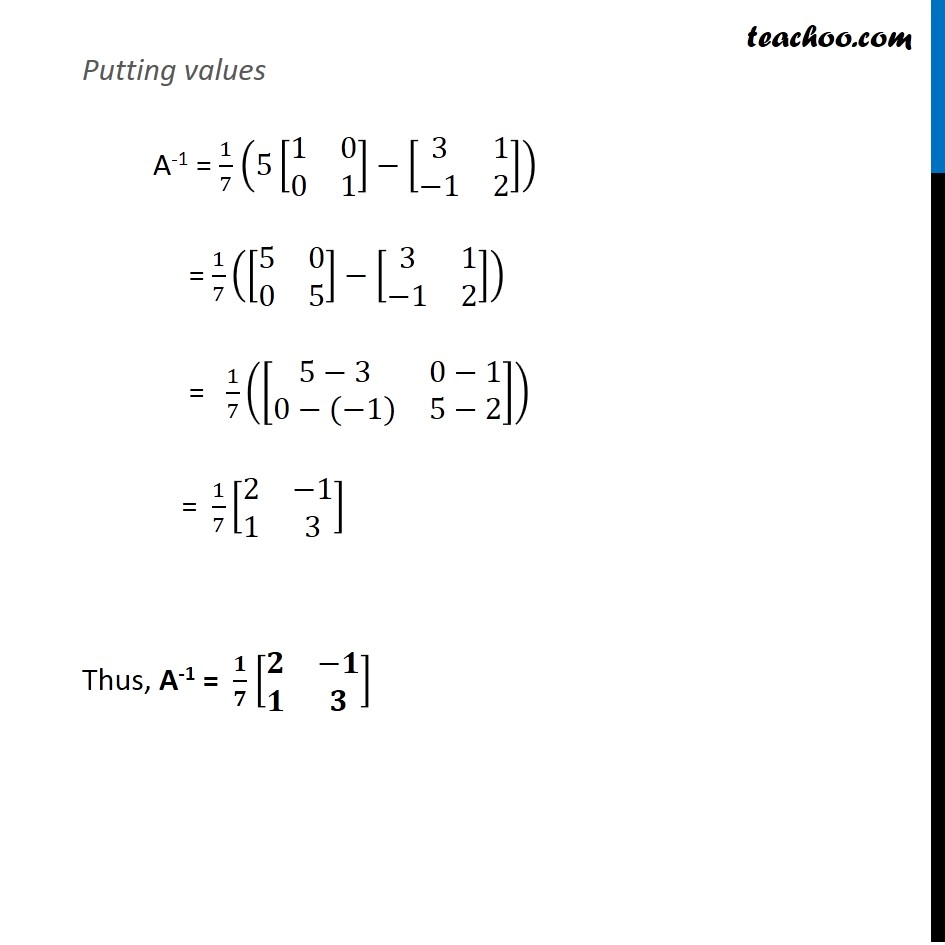1. Chapter 4 Class 12 Determinants (Term 1)
2. Concept wise
3. Finding inverse when Equation of matrice given

Transcript

Ex 4.5, 13 If A = [■8(3&1@−1&2)] show that A2 – 5A + 7I = O. Hence find A–1. Calculating A2 A2 = A.A = [■8(3&1@−1&2)] [■8(3&1@−1&2)] =[■8(3(3)+1(−1)&3(1)+1(2)@−1(3)+2(−1)&−1(1)+2(2))] = [■8(9−1&3+2@−3−2&−1+4)] = [■8(8&5@−5&3)] Solving L.H.S A2 – 5A + 7I = [■8(8&5@−5&3)] – 5 [■8(3&1@−1&2)] + 7 [■8(1&0@0&1)] = [■8(8&5@−5&3)] – [■8(5(3)&5(1)@5(−1)&5(2))] + [■8(7(1)&7(0)@7(0)&7(1))] = [■8(8&5@−5&3)] – [■8(15&5@−5&10)] + [■8(7&0@0&7)] = [■8(8−15+7&5−5+0@−5−(−5)+0&3−10+7)] = [■8(8−15+7&5−5+0@−5+5+0&3−10+7)] = [■8(0&0@0&0)] = O Thus, A2 – 5A + 7I = O Hence proved Finding A–1 A2 – 5A + 7I = O Pre multiplying A-1 both sides A-1 (A2 – 5A + 7I ) = A-1 O A-1 . A2 – 5A-1A + 7A-1 = O A-1 AA – 5(A-1 A) + 7A-1 = O (A-1A)A – 5 (A-1 A) + 7 (A-1 I) = O IA – 5I + 7A-1 = O A – 5I + 7 A-1 = 0 7A-1 = 5I – A A-1 = 𝟏/𝟕 (5I – A) (AA-1 = I ) (IA = A) Putting values A-1 = 1/7 (5[■8(1&0@0&1)]−[■8(3&1@−1&2)]) = 1/7 ([■8(5&0@0&5)]−[■8(3&1@−1&2)]) = 1/7 ([■8(5−3&0−1@0−(−1)&5−2)]) = 1/7 [■8(2&−1@1&3)] Thus, A-1 = 𝟏/𝟕 [■8(𝟐&−𝟏@𝟏&𝟑)]

Finding inverse when Equation of matrice given

Chapter 4 Class 12 Determinants (Term 1)
Concept wise